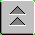[Index]

# TASS

DIRECTIVITY ADVANTAGE OF LARGE APERTURE ARRAYS

It has long been known that low frequencies propagation substantially further than high frequencies. The attenuation is acoustic signals depends on the square frequency. Mathmatically speaking dB attenuation = ar, where

r = range (m)

a = attenuation coefficient.

By comparison, we shall see what this statement means. Neglecting all other considerations, consider the attenuation of a typical 2000 Hz signal compared to a 50 Hz signal.

a = 0.9 dB/m at 2000 Hz

a = 0.01 dB/m at 50 Hz

Assuming a nominal FOM of 100 dB, that means at the signal would be lost at a range of

r = 100 m for 2000 Hz but

r = 10, 000 m for 50 Hz.

Beyond this is even more amazing, at 10 Hz, the range would be 100, 000m! So it is abundantly clear that the best detection ranges apply to the lowest frequencies. Why then aren't all sonar systems designed to work at these low frequencies? The answer is diffraction. A diffraction limited hydrophone (or system of hydrophones) has a beamwidth that goes approximately as q = l/l, where l is the size of the array )or aperture. So for low frequencies, the beamwidth starts to get very large, for large wavelengths. Unless the aperture is also large, the beamwidth becomes unacceptably large. This has two direct effects.

The first beamwidth problem is the ability to measure a bearing. Bearing accuracy depends on a well defined beam. As the beam becomes large, then it becomes impossible to measure the bearing to the source. Secondly, the sonar system becomes less sensitive. It cannot exclude noise by using its directionality. This is expressed by the term DI (directivity index) in the basic sonar equations. In other words, DI becomes very low for sonar systems at low frequencies

The solution to both these problems is a large aperture array. An example is the towed array sonar systems (TASS). Because the aperture is large, the bearing accuracy is improved and the DI becomes large again. It is not uncommon to see DI of >30 dB at these frequencies. The result is superior performance in an environment with very low propagation losses. In general, TASS detection ranges are enormous. In fact, they are so sensitive that low frequency background noise (shipping) can be heard for hundreds of miles, often making it difficult to pick out interesting signals from the plethura of sonar contacts.